Constituent Parsing as Sequence Labeling# 树的序列化

## 编码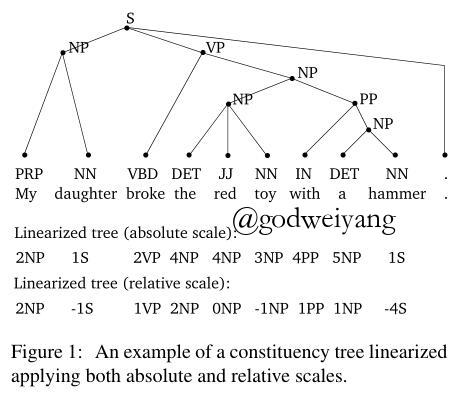$k$叉树编码：如果句法树所有产生式全部是$k$叉的，那么还可以将编码进一步简化，具体做法就是将所有的负数$n_i$统一为一个负数就行。为什么这里就不需要对负数进行区分了呢？这还得从句法树的解码说起，我们看一看是怎么从序列解码成句法树的。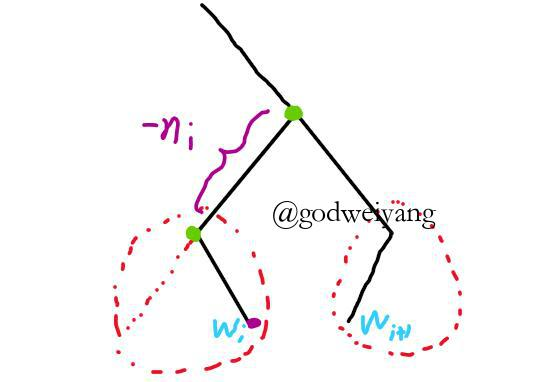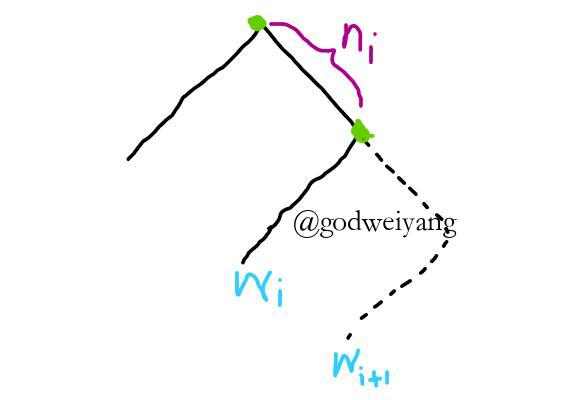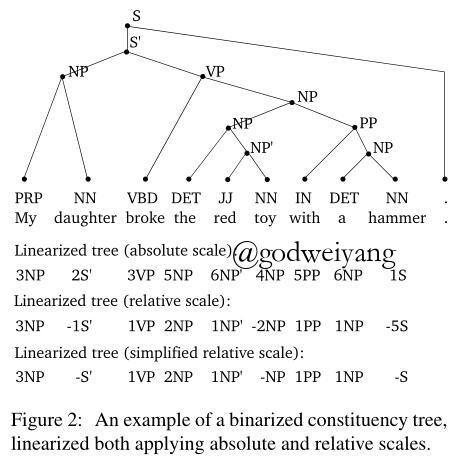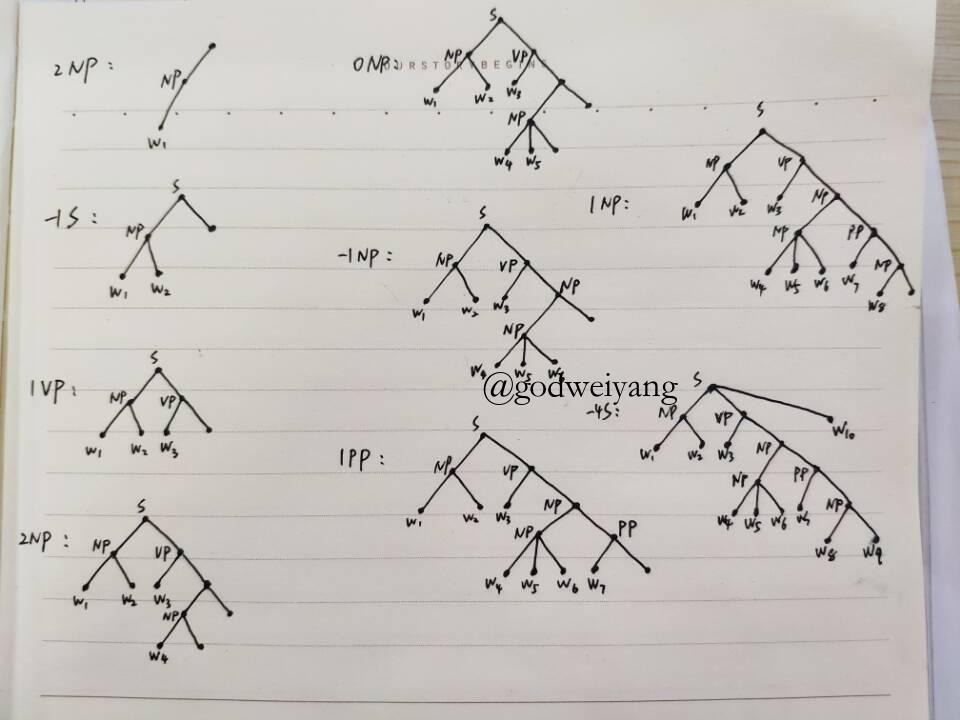## 理论证明

$\alpha_0 (\bullet_1) \alpha_1 (\bullet_2) \ldots \alpha_{\left| w \right| - 1} (\bullet_{\left| w \right|}) \alpha_{\left| w \right|}$

$\alpha_{0)} \alpha_{0(} (\bullet_1) \alpha_{1)} \alpha_{1(} (\bullet_2) \ldots (\bullet_{\left| w \right|}) \alpha_{\left| w \right|)} \alpha_{\left| w \right|(}$

$\beta_1 \beta_2 \ldots \beta_{\left| w \right|}$

$\delta(\beta_1)\delta(\beta_2)\ldots\delta(\beta_{\left|w\right| - 1})$

## 限制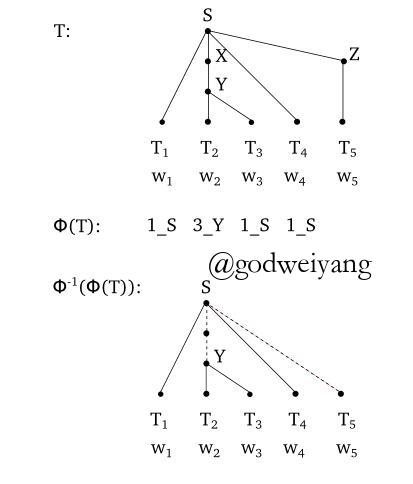# 实验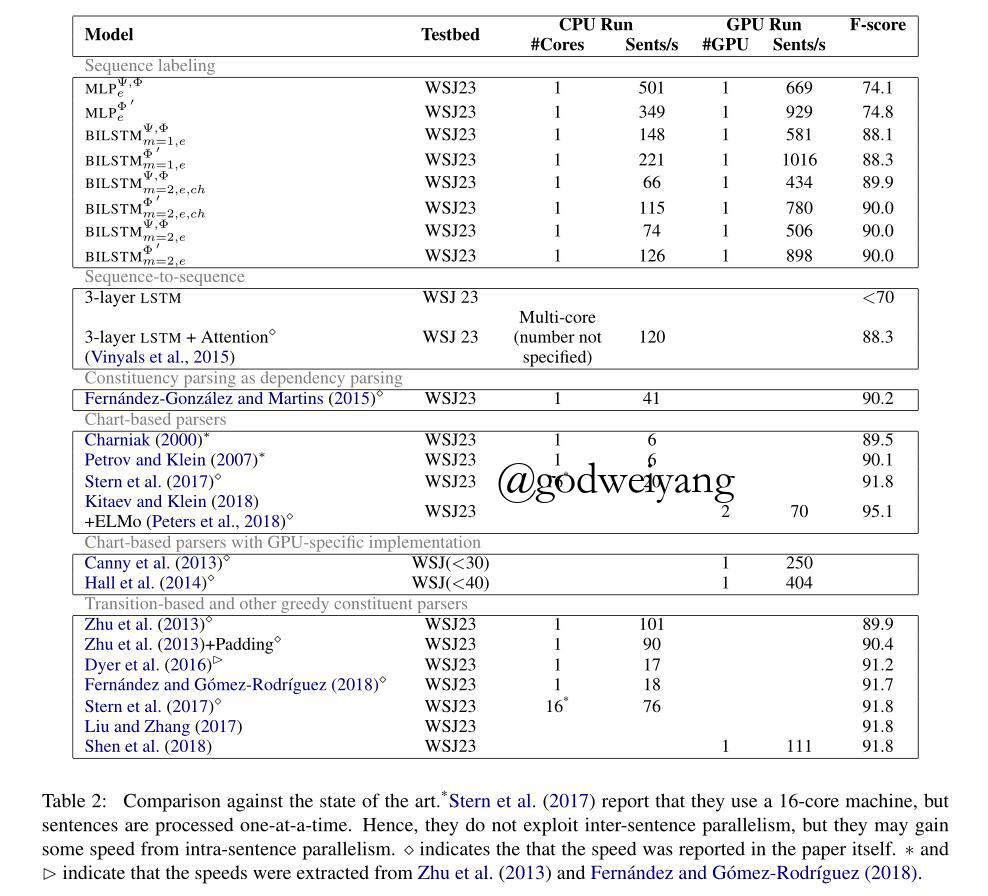# 结论与展望

转载规则

《Constituent Parsing as Sequence Labeling》 韦阳 采用 知识共享署名 4.0 国际许可协议 进行许可。
上一篇Better, Faster, Stronger Sequence Tagging Constituent Parsers

2019-03-11Latex撰写论文常用技巧总结

2019-01-13
目录